# Infiltration and drainage test descriptions

## The 2-phase analytic infiltration solution

The physical setup studied in this section is a 1D column that is initially unsaturated, and which is subject to a constant injection of fluid from its top. This is of physical importance because it is a model of constant rainfall recharge to an initially dry groundwater system. The top surface becomes saturated, and this saturated zone moves downwards into the column, diffusing as it goes. The problem is of computational interest because under certain conditions an analytic solution is available for the saturation profile as a function of depth and time.

The Richards' equation for an incompressible fluid in one spatial dimension () reads (1) where (2) and (3) Here which is the capillary pressure, and recall that .

The analytic solution of this nonlinear diffusion-advection relevant to constant infiltration to groundwater has been derived by Broadbridge and White (Broadbridge and White, 1988) for certain functions and . Broadbridge and White assume the hydraulic conductivity is (4) where (5) and the parameters obey , , and . The diffusivity is of the form . This leads to very complicated relationships between the capillary pressure, , and the saturation, except in the case where is small, when they are related through (6) with being the final parameter introduced by Broadbridge and White.

Broadbridge and White derive time-dependent solutions for constant recharge to one end of a semi-infinite line. Their solutions are quite lengthy, will not be written here. To compare with MOOSE, the following parameters are used — the hydraulic parameters are those used in Figure 3 of Broadbridge and White:

Table 1: Parameter values used in the 2-phase tests

ParameterValue
Bar length20m
Bar porosity0.25
Bar permeability1
Gravity0.1m.s
Fluid density10kg.m
Fluid viscosity4Pa.s
0m.s
1m.s
0m.s
1m.s
1.5
2Pa
Recharge rate 0.5

Broadbridge and white consider the case where the initial condition is , but this yields , which is impossible to use in a MOOSE model. Therefore the initial condition Pa is used which avoids any underflow problems. The recharge rate of corresponds in the MOOSE model to a recharge rate of kg.m.s. Note that m.s, so that the and may be encoded as and in the relative permeability function Eq. (4) in a straightforward way.

Figure 1 shows good agreement between the analytic solution of Broadbridge and White and the MOOSE implementation. There are minor discrepancies for small values of saturation: these get smaller as the temporal and spatial resolution is increased, but never totally disappear due to the initial condition of Pa.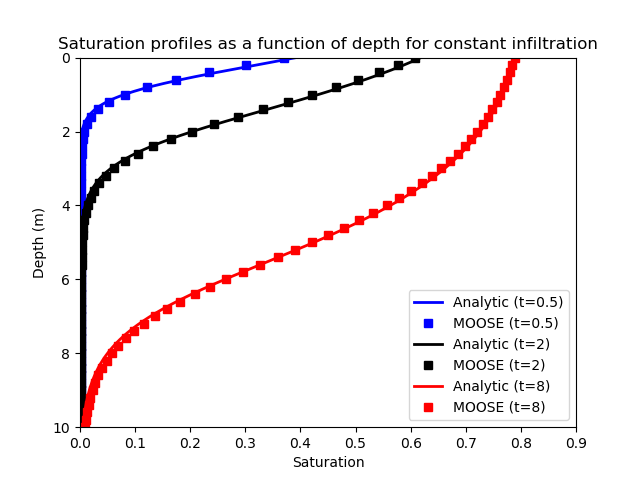Figure 1: Comparison of the Broadbridge and White analytical solution with the MOOSE solution for 3 times. This figure is shown in the standard format used in the Broadbridge-White paper: the constant recharge is applied to the top (where depth is zero) and gravity acts downwards in this figure.

Two tests are part of the automatic test suite (one is marked "heavy" because it is a high-resolution version).

[Mesh]
type = GeneratedMesh
dim = 2
nx = 400
ny = 1
xmin = -10
xmax = 10
ymin = 0
ymax = 0.05
[]

[GlobalParams]
PorousFlowDictator = dictator
[]

[Functions]
[./dts]
type = PiecewiseLinear
y = '1E-5 1E-2 1E-2 1E-1'
x = '0 1E-5 1 10'
[../]
[]

[UserObjects]
[./dictator]
type = PorousFlowDictator
porous_flow_vars = pressure
number_fluid_phases = 1
number_fluid_components = 1
[../]
[./pc]
type = PorousFlowCapillaryPressureBW
Sn = 0.0
Ss = 1.0
C = 1.5
las = 2
[../]
[]

[Modules]
[./FluidProperties]
[./simple_fluid]
type = SimpleFluidProperties
bulk_modulus = 2e9
viscosity = 4
density0 = 10
thermal_expansion = 0
[../]
[../]
[]

[Materials]
[./massfrac]
type = PorousFlowMassFraction
[../]
[./temperature]
type = PorousFlowTemperature
[../]
[./simple_fluid]
type = PorousFlowSingleComponentFluid
fp = simple_fluid
phase = 0
[../]
[./ppss]
type = PorousFlow1PhaseP
porepressure = pressure
capillary_pressure = pc
[../]
[./relperm]
type = PorousFlowRelativePermeabilityBW
Sn = 0.0
Ss = 1.0
Kn = 0
Ks = 1
C = 1.5
phase = 0
[../]
[./porosity]
type = PorousFlowPorosityConst
porosity = 0.25
[../]
[./permeability]
type = PorousFlowPermeabilityConst
permeability = '1 0 0  0 1 0  0 0 1'
[../]
[]

[Variables]
[./pressure]
initial_condition = -9E2
[../]
[]

[Kernels]
[./mass0]
type = PorousFlowMassTimeDerivative
fluid_component = 0
variable = pressure
[../]
[./flux0]
type = PorousFlowAdvectiveFlux
fluid_component = 0
variable = pressure
gravity = '-0.1 0 0'
[../]
[]

[AuxVariables]
[./SWater]
family = MONOMIAL
order = CONSTANT
[../]
[]

[AuxKernels]
[./SWater]
type = MaterialStdVectorAux
property = PorousFlow_saturation_qp
index = 0
variable = SWater
[../]
[]

[BCs]
[./recharge]
type = PorousFlowSink
variable = pressure
boundary = right
flux_function = -1.25 # corresponds to Rstar being 0.5 because i have to multiply by density*porosity
[../]
[]

[Preconditioning]
[./andy]
type = SMP
full = true
petsc_options = '-snes_converged_reason -ksp_diagonal_scale -ksp_diagonal_scale_fix -ksp_gmres_modifiedgramschmidt -snes_linesearch_monitor'
petsc_options_iname = '-ksp_type -pc_type -sub_pc_type -sub_pc_factor_shift_type -pc_asm_overlap -snes_atol -snes_rtol -snes_max_it'
petsc_options_value = 'gmres      asm      lu           NONZERO                   2               1E-10      1E-10      10000'
[../]
[]

[VectorPostprocessors]
[./swater]
type = LineValueSampler
variable = SWater
start_point = '-10 0 0'
end_point = '10 0 0'
sort_by = x
num_points = 101
execute_on = timestep_end
[../]
[]

[Executioner]
type = Transient
solve_type = Newton
petsc_options = '-snes_converged_reason'
end_time = 8

[./TimeStepper]
type = FunctionDT
function = dts
[../]
[]

[Outputs]
file_base = bw01
sync_times = '0.5 2 8'
[./exodus]
type = Exodus
sync_only = true
[../]
[./along_line]
type = CSV
sync_only = true
[../]
[]

(modules/porous_flow/test/tests/infiltration_and_drainage/bw01.i)

## The two-phase analytic drainage solution

Warrick, Lomen and Islas (Warrick et al., 1990) extended the analysis of Broadbridge and White to include the case of drainage from a medium.

The setup is an initially-saturated semi-infinite column of material that drains freely from its lower end. This is simulated by placing a boundary condition of at the lower end. To obtain their analytical solutions, Warrick, Lomen and Islas make the same assumptions as Broadbridge and White concerning the diffusivity and conductivity of the medium. Their solutions are quite lengthy, so are not written here

A MOOSE model with the parameters almost identical to those listed in Table 1 is compared with the analytical solutions. The only differences are that the "bar" length is m (to avoid any interference from the lower Dirichlet boundary condition), and since there is no recharge. The initial condition is Pa: the choice leads to poor convergence since by construction the Broadbridge-White capillary function is only designed to simulate the unsaturated zone and a sensible extension to is discontinuous at .

Figure 2 shows good agreement between the analytic solution and the MOOSE implementation. Any minor discrepancies get smaller as the temporal and spatial resolution increase.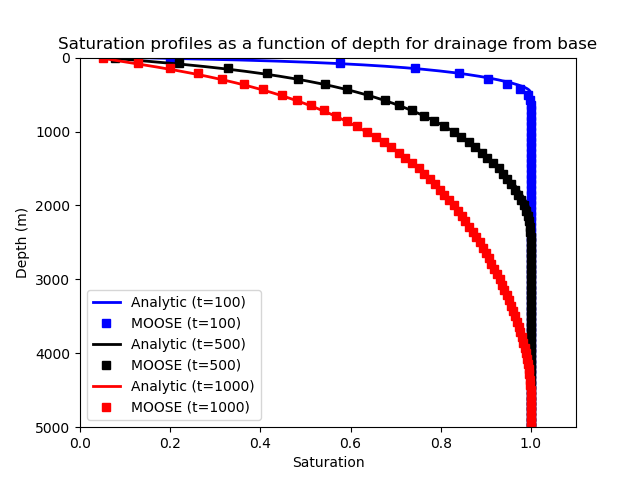Figure 2: Comparison of the Warrick, Lomen and Islas analytical solution with the MOOSE solution for 3 times. This figure is shown in the standard format used in the literature: the top of the model is at the top of the figure, and gravity acts downwards in this figure, with fluid draining from the infinitely deep point.

Two tests are part of the automatic test suite (one is marked "heavy" because it is a high-resolution version).

[Mesh]
type = GeneratedMesh
dim = 2
nx = 1000
ny = 1
xmin = -10000
xmax = 0
ymin = 0
ymax = 0.05
[]

[GlobalParams]
PorousFlowDictator = dictator
[]

[UserObjects]
[./dictator]
type = PorousFlowDictator
porous_flow_vars = pressure
number_fluid_phases = 1
number_fluid_components = 1
[../]
[./pc]
type = PorousFlowCapillaryPressureBW
Sn = 0.0
Ss = 1.0
C = 1.5
las = 2
[../]
[]

[Modules]
[./FluidProperties]
[./simple_fluid]
type = SimpleFluidProperties
bulk_modulus = 2e9
viscosity = 4
density0 = 10
thermal_expansion = 0
[../]
[../]
[]

[Materials]
[./massfrac]
type = PorousFlowMassFraction
[../]
[./temperature]
type = PorousFlowTemperature
[../]
[./simple_fluid]
type = PorousFlowSingleComponentFluid
fp = simple_fluid
phase = 0
[../]
[./ppss]
type = PorousFlow1PhaseP
porepressure = pressure
capillary_pressure = pc
[../]
[./relperm]
type = PorousFlowRelativePermeabilityBW
Sn = 0.0
Ss = 1.0
Kn = 0
Ks = 1
C = 1.5
phase = 0
[../]
[./porosity]
type = PorousFlowPorosityConst
porosity = 0.25
[../]
[./permeability]
type = PorousFlowPermeabilityConst
permeability = '1 0 0  0 1 0  0 0 1'
[../]
[]

[Variables]
[./pressure]
initial_condition = -1E-4
[../]
[]

[Kernels]
[./mass0]
type = PorousFlowMassTimeDerivative
fluid_component = 0
variable = pressure
[../]
[./flux0]
type = PorousFlowAdvectiveFlux
fluid_component = 0
variable = pressure
gravity = '-0.1 0 0'
[../]
[]

[AuxVariables]
[./SWater]
family = MONOMIAL
order = CONSTANT
[../]
[]

[AuxKernels]
[./SWater]
type = MaterialStdVectorAux
property = PorousFlow_saturation_qp
index = 0
variable = SWater
[../]
[]

[BCs]
[./base]
type = DirichletBC
boundary = 'left'
value = -1E-4
variable = pressure
[../]
[]

[Preconditioning]
[./andy]
type = SMP
full = true
petsc_options = '-snes_converged_reason -ksp_diagonal_scale -ksp_diagonal_scale_fix -ksp_gmres_modifiedgramschmidt -snes_linesearch_monitor'
petsc_options_iname = '-ksp_type -pc_type -sub_pc_type -sub_pc_factor_shift_type -pc_asm_overlap -snes_atol -snes_rtol -snes_max_it'
petsc_options_value = 'gmres      asm      lu           NONZERO                   2               1E-10      1E-10      10000'
[../]
[]

[VectorPostprocessors]
[./swater]
type = LineValueSampler
variable = SWater
start_point = '-5000 0 0'
end_point = '0 0 0'
sort_by = x
num_points = 71
execute_on = timestep_end
[../]
[]

[Executioner]
type = Transient
solve_type = Newton
petsc_options = '-snes_converged_reason'
end_time = 1000
dt = 1
[]

[Outputs]
file_base = wli01
sync_times = '100 500 1000'
[./exodus]
type = Exodus
sync_only = true
[../]
[./along_line]
type = CSV
sync_only = true
[../]
[]

(modules/porous_flow/test/tests/infiltration_and_drainage/wli01.i)

## Single-phase infiltration and drainage

Forsyth, Wu and Pruess (Forsyth et al., 1995) describe a HYDRUS simulation of an experiment involving infiltration (experiment 1) and subsequent drainage (experiment 2) in a large caisson. The simulation is effectively one dimensional, and is shown in Figure 3.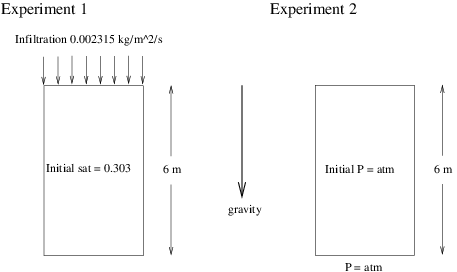Figure 3: Two experimental setups from Forsyth, Wu and Pruess. Experiment 1 involves infiltration of water into an initially unsaturated caisson. Experiment 2 involves drainage of water from an initially saturated caisson.

The properties common to each experiment are listed in Table 2

Table 2: Parameter values used in the single-phase infiltration and drainage tests

ParameterValue
Caisson porosity0.33
Caisson permeabilitym
Gravity10m.s
Water density at STP1000kg.m
Water viscosity0.00101Pa.s
Water bulk modulus20MPa
Water residual saturation0.0
Air residual saturation0.0
Air pressure0.0
van Genuchten Pa
van Genuchten 0.336
van Genuchten turnover0.99

In each experiment 120 finite elements are used along the length of the Caisson. The modified van-Genuchten relative permeability curve with a "turnover" (set at ) is employed in order to improve convergence significantly. Hydrus also uses a modified van-Genuchten curve, although I couldn't find any details on the modification.

In experiment 1, the caisson is initially at saturation 0.303 (Pa), and water is pumped into the top with a rate 0.002315kg.m.s. This causes a front of water to advance down the caisson. Figure 4 shows the agreement between MOOSE and the published result (this result was obtained by extracting data by hand from online graphics).

In experiment 2, the caisson is initially fully saturated at , and the bottom is held at to cause water to drain via the action of gravity. Figure 4 and Figure 5 show the agreement between MOOSE and the published result.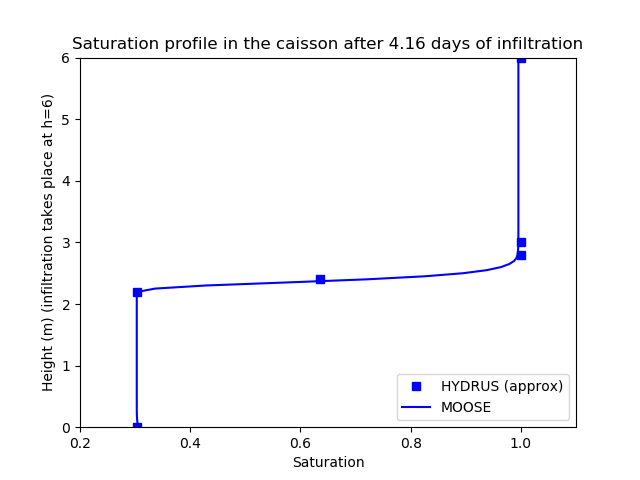Figure 4: Saturation profile in the caisson after 4.16 days of infiltration. Note that the HYDRUS results are only approximate they were extrated by hand from online graphics.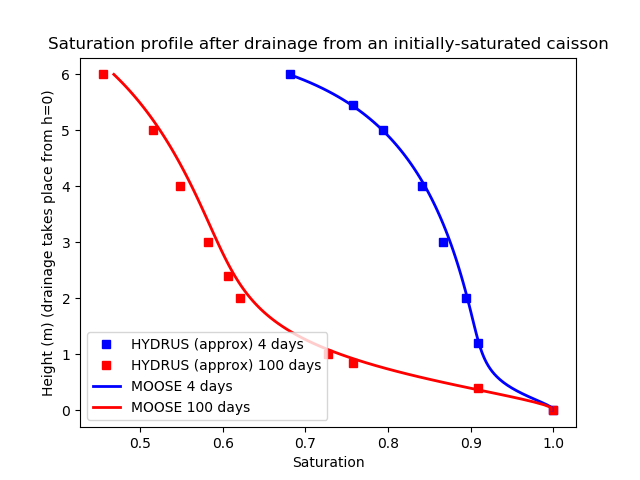Figure 5: Saturation profiles in the caisson after drainage from an initially-saturated simulation (4 days and 100 days profiles). Note that the HYDRUS results are only approximate they were extrated by hand from online graphics.

Experiment 1 and the first 4 simulation days of experiment 2 are marked as "heavy" in the PorousFlow test suite since the simulations take around 3 seconds to complete.

The input file for Experiment 1:

[Mesh]
type = GeneratedMesh
dim = 2
nx = 120
ny = 1
xmin = 0
xmax = 6
ymin = 0
ymax = 0.05
[]

[GlobalParams]
PorousFlowDictator = dictator
[]

[Functions]
[./dts]
type = PiecewiseLinear
y = '1E-2 1 10 500 5000 5000'
x = '0 10 100 1000 10000 100000'
[../]
[]

[UserObjects]
[./dictator]
type = PorousFlowDictator
porous_flow_vars = pressure
number_fluid_phases = 1
number_fluid_components = 1
[../]
[./pc]
type = PorousFlowCapillaryPressureVG
m = 0.336
alpha = 1.43e-4
[../]
[]

[Modules]
[./FluidProperties]
[./simple_fluid]
type = SimpleFluidProperties
bulk_modulus = 2e7
viscosity = 1.01e-3
density0 = 1000
thermal_expansion = 0
[../]
[../]
[]

[Materials]
[./massfrac]
type = PorousFlowMassFraction
[../]
[./temperature]
type = PorousFlowTemperature
[../]
[./simple_fluid]
type = PorousFlowSingleComponentFluid
fp = simple_fluid
phase = 0
[../]
[./ppss]
type = PorousFlow1PhaseP
porepressure = pressure
capillary_pressure = pc
[../]
[./relperm]
type = PorousFlowRelativePermeabilityVG
m = 0.336
seff_turnover = 0.99
phase = 0
[../]
[./porosity]
type = PorousFlowPorosityConst
porosity = 0.33
[../]
[./permeability]
type = PorousFlowPermeabilityConst
permeability = '0.295E-12 0 0  0 0.295E-12 0  0 0 0.295E-12'
[../]
[]

[Variables]
[./pressure]
initial_condition = -72620.4
[../]
[]

[Kernels]
[./mass0]
type = PorousFlowMassTimeDerivative
fluid_component = 0
variable = pressure
[../]
[./flux0]
type = PorousFlowAdvectiveFlux
fluid_component = 0
variable = pressure
gravity = '-10 0 0'
[../]
[]

[AuxVariables]
[./SWater]
family = MONOMIAL
order = CONSTANT
[../]
[]

[AuxKernels]
[./SWater]
type = MaterialStdVectorAux
property = PorousFlow_saturation_qp
index = 0
variable = SWater
[../]
[]

[BCs]
[./base]
type = PorousFlowSink
boundary = right
flux_function = -2.315E-3
variable = pressure
[../]
[]

[Preconditioning]
[./andy]
type = SMP
full = true
petsc_options = '-snes_converged_reason -ksp_diagonal_scale -ksp_diagonal_scale_fix -ksp_gmres_modifiedgramschmidt -snes_linesearch_monitor'
petsc_options_iname = '-ksp_type -pc_type -sub_pc_type -sub_pc_factor_shift_type -pc_asm_overlap -snes_atol -snes_rtol -snes_max_it'
petsc_options_value = 'gmres      asm      lu           NONZERO                   2               1E-10      1E-10      10'
[../]
[]

[VectorPostprocessors]
[./swater]
type = LineValueSampler
variable = SWater
start_point = '0 0 0'
end_point = '6 0 0'
sort_by = x
num_points = 121
execute_on = timestep_end
[../]
[]

[Executioner]
type = Transient
solve_type = Newton
petsc_options = '-snes_converged_reason'
end_time = 359424

[./TimeStepper]
type = FunctionDT
function = dts
[../]
[]

[Outputs]
file_base = rd01
[./exodus]
type = Exodus
execute_on = final
[../]
[./along_line]
type = CSV
execute_on = final
[../]
[]

(modules/porous_flow/test/tests/infiltration_and_drainage/rd01.i)

The input file for the first 4 simulation days of Experiment 2:

[Mesh]
type = GeneratedMesh
dim = 2
nx = 120
ny = 1
xmin = 0
xmax = 6
ymin = 0
ymax = 0.05
[]

[GlobalParams]
PorousFlowDictator = dictator
[]

[Functions]
[./dts]
type = PiecewiseLinear
y = '1E-2 1 10 500 5000 50000'
x = '0 10 100 1000 10000 500000'
[../]
[]

[UserObjects]
[./dictator]
type = PorousFlowDictator
porous_flow_vars = pressure
number_fluid_phases = 1
number_fluid_components = 1
[../]
[./pc]
type = PorousFlowCapillaryPressureVG
m = 0.336
alpha = 1.43e-4
[../]
[]

[Modules]
[./FluidProperties]
[./simple_fluid]
type = SimpleFluidProperties
bulk_modulus = 2e7
viscosity = 1.01e-3
density0 = 1000
thermal_expansion = 0
[../]
[../]
[]

[Materials]
[./massfrac]
type = PorousFlowMassFraction
[../]
[./temperature]
type = PorousFlowTemperature
[../]
[./simple_fluid]
type = PorousFlowSingleComponentFluid
fp = simple_fluid
phase = 0
[../]
[./ppss]
type = PorousFlow1PhaseP
porepressure = pressure
capillary_pressure = pc
[../]
[./relperm]
type = PorousFlowRelativePermeabilityVG
m = 0.336
seff_turnover = 0.99
phase = 0
[../]
[./porosity]
type = PorousFlowPorosityConst
porosity = 0.33
[../]
[./permeability]
type = PorousFlowPermeabilityConst
permeability = '0.295E-12 0 0  0 0.295E-12 0  0 0 0.295E-12'
[../]
[]

[Variables]
[./pressure]
initial_condition = 0.0
[../]
[]

[Kernels]
[./mass0]
type = PorousFlowMassTimeDerivative
fluid_component = 0
variable = pressure
[../]
[./flux0]
type = PorousFlowAdvectiveFlux
fluid_component = 0
variable = pressure
gravity = '-10 0 0'
[../]
[]

[AuxVariables]
[./SWater]
family = MONOMIAL
order = CONSTANT
[../]
[]

[AuxKernels]
[./SWater]
type = MaterialStdVectorAux
property = PorousFlow_saturation_qp
index = 0
variable = SWater
[../]
[]

[BCs]
[./base]
type = DirichletBC
boundary = left
value = 0.0
variable = pressure
[../]
[]

[Preconditioning]
[./andy]
type = SMP
full = true
petsc_options = '-snes_converged_reason -ksp_diagonal_scale -ksp_diagonal_scale_fix -ksp_gmres_modifiedgramschmidt -snes_linesearch_monitor'
petsc_options_iname = '-ksp_type -pc_type -sub_pc_type -sub_pc_factor_shift_type -pc_asm_overlap -snes_atol -snes_rtol -snes_max_it'
petsc_options_value = 'gmres      asm      lu           NONZERO                   2               1E-10      1E-10      10'
[../]
[]

[VectorPostprocessors]
[./swater]
type = LineValueSampler
variable = SWater
start_point = '0 0 0'
end_point = '6 0 0'
sort_by = x
num_points = 121
execute_on = timestep_end
[../]
[]

[Executioner]
type = Transient
solve_type = Newton
petsc_options = '-snes_converged_reason'
end_time = 345600

[./TimeStepper]
type = FunctionDT
function = dts
[../]
[]

[Outputs]
file_base = rd02
[./exodus]
type = Exodus
execute_on = final
[../]
[./along_line]
type = CSV
execute_on = final
[../]
[]

(modules/porous_flow/test/tests/infiltration_and_drainage/rd02.i)

The input file for the latter 96 days of Experiment 2:

[Mesh]
file = gold/rd02.e
[]

[GlobalParams]
PorousFlowDictator = dictator
[]

[Functions]
[./dts]
type = PiecewiseLinear
y = '2E4 1E6'
x = '0 1E6'
[../]
[]

[UserObjects]
[./dictator]
type = PorousFlowDictator
porous_flow_vars = pressure
number_fluid_phases = 1
number_fluid_components = 1
[../]
[./pc]
type = PorousFlowCapillaryPressureVG
m = 0.336
alpha = 1.43e-4
[../]
[]

[Modules]
[./FluidProperties]
[./simple_fluid]
type = SimpleFluidProperties
bulk_modulus = 2e7
viscosity = 1.01e-3
density0 = 1000
thermal_expansion = 0
[../]
[../]
[]

[Materials]
[./massfrac]
type = PorousFlowMassFraction
[../]
[./temperature]
type = PorousFlowTemperature
[../]
[./simple_fluid]
type = PorousFlowSingleComponentFluid
fp = simple_fluid
phase = 0
[../]
[./ppss]
type = PorousFlow1PhaseP
porepressure = pressure
capillary_pressure = pc
[../]
[./relperm]
type = PorousFlowRelativePermeabilityVG
m = 0.336
seff_turnover = 0.99
phase = 0
[../]
[./porosity]
type = PorousFlowPorosityConst
porosity = 0.33
[../]
[./permeability]
type = PorousFlowPermeabilityConst
permeability = '0.295E-12 0 0  0 0.295E-12 0  0 0 0.295E-12'
[../]
[]

[Variables]
[./pressure]
initial_from_file_timestep = 1
initial_from_file_var = pressure
[../]
[]

[Kernels]
[./mass0]
type = PorousFlowMassTimeDerivative
fluid_component = 0
variable = pressure
[../]
[./flux0]
type = PorousFlowAdvectiveFlux
fluid_component = 0
variable = pressure
gravity = '-10 0 0'
[../]
[]

[AuxVariables]
[./SWater]
family = MONOMIAL
order = CONSTANT
[../]
[]

[AuxKernels]
[./SWater]
type = MaterialStdVectorAux
property = PorousFlow_saturation_qp
index = 0
variable = SWater
[../]
[]

[BCs]
[./base]
type = DirichletBC
boundary = left
value = 0.0
variable = pressure
[../]
[]

[Preconditioning]
[./andy]
type = SMP
full = true
petsc_options = '-snes_converged_reason -ksp_diagonal_scale -ksp_diagonal_scale_fix -ksp_gmres_modifiedgramschmidt -snes_linesearch_monitor'
petsc_options_iname = '-ksp_type -pc_type -sub_pc_type -sub_pc_factor_shift_type -pc_asm_overlap -snes_atol -snes_rtol -snes_max_it'
petsc_options_value = 'gmres      asm      lu           NONZERO                   2               1E-10      1E-10      10'
[../]
[]

[VectorPostprocessors]
[./swater]
type = LineValueSampler
variable = SWater
start_point = '0 0 0'
end_point = '6 0 0'
sort_by = x
num_points = 121
execute_on = timestep_end
[../]
[]

[Executioner]
type = Transient
solve_type = Newton
petsc_options = '-snes_converged_reason'
end_time = 8.2944E6

[./TimeStepper]
type = FunctionDT
function = dts
[../]
[]

[Outputs]
file_base = rd03
[./exodus]
type = Exodus
execute_on = 'initial final'
[../]
[./along_line]
type = CSV
execute_on = final
[../]
[]

(modules/porous_flow/test/tests/infiltration_and_drainage/rd03.i)

## Water infiltration into a two-phase (oil-water) system

An analytic solution of the two-phase Richards' equations with gravity on a semi-infinite line , with a constant water infiltration flux at has been derived by (Rogers et al., 1983) (Unfortunately there must be a typo in the RSC paper as for nonzero gravity their results are clearly incorrect.). The authors assume incompressible fluids; linear relative permeability relationships; the "oil" (or "gas") viscosity is larger than the water viscosity; and, a certain functional form for the capillary pressure. When the oil viscosity is exactly twice the water viscosity, their effective saturation reads (7) where is the capillary pressure, and and are arbitrary parameters to be defined by the user in the PorousFlow implementation. For other oil/water viscosity ratios is more complicated, and note that their formulation allows , but only the particular form Eq. (7) need be used to validate the MOOSE implementation.

RSC's solutions are quite lengthy, so I will not write them here. To compare with MOOSE, the following parameters are used:

Table 3: Parameter values used in the Rogers-Stallybrass-Clements infiltration tests

ParameterValue
Bar length10 m
Bar porosity0.25
Bar permeabilitym
Gravity0 m.s
Water density10 kg.m
Water viscosityPa.s
Oil density20 kg.m
Oil viscosityPa.s
Capillary 10 Pa
Capillary 1 Pa
Initial water pressure0 Pa
Initial oil pressure15 Pa
Initial water saturation0.08181
Initial oil saturation0.91819
Water injection rate1 kg.s.m

The input file:

# RSC test with high-res time and spatial resolution
[Mesh]
type = GeneratedMesh
dim = 2
nx = 600
ny = 1
xmin = 0
xmax = 10 # x is the depth variable, called zeta in RSC
ymin = 0
ymax = 0.05
[]

[GlobalParams]
PorousFlowDictator = dictator
gravity = '0 0 0'
[]

[Functions]
[./dts]
type = PiecewiseLinear
y = '3E-3 3E-2 0.05'
x = '0 1 5'
[../]
[]

[UserObjects]
[./dictator]
type = PorousFlowDictator
porous_flow_vars = 'pwater poil'
number_fluid_phases = 2
number_fluid_components = 2
[../]
[./pc]
type = PorousFlowCapillaryPressureRSC
oil_viscosity = 2E-3
scale_ratio = 2E3
shift = 10
[../]
[]

[Modules]
[./FluidProperties]
[./water]
type = SimpleFluidProperties
bulk_modulus = 2e9
density0 = 10
thermal_expansion = 0
viscosity = 1e-3
[../]
[./oil]
type = SimpleFluidProperties
bulk_modulus = 2e9
density0 = 20
thermal_expansion = 0
viscosity = 2e-3
[../]
[../]
[]

[Materials]
[./temperature]
type = PorousFlowTemperature
[../]
[./ppss]
type = PorousFlow2PhasePP
phase0_porepressure = pwater
phase1_porepressure = poil
capillary_pressure = pc
[../]
[./massfrac]
type = PorousFlowMassFraction
mass_fraction_vars = 'massfrac_ph0_sp0 massfrac_ph1_sp0'
[../]
[./water]
type = PorousFlowSingleComponentFluid
fp = water
phase = 0
compute_enthalpy = false
compute_internal_energy = false
[../]
[./oil]
type = PorousFlowSingleComponentFluid
fp = oil
phase = 1
compute_enthalpy = false
compute_internal_energy = false
[../]
[./relperm_water]
type = PorousFlowRelativePermeabilityCorey
n = 1
phase = 0
[../]
[./relperm_oil]
type = PorousFlowRelativePermeabilityCorey
n = 1
phase = 1
[../]
[./porosity]
type = PorousFlowPorosityConst
porosity = 0.25
[../]
[./permeability]
type = PorousFlowPermeabilityConst
permeability = '1E-5 0 0  0 1E-5 0  0 0 1E-5'
[../]
[]

[Variables]
[./pwater]
[../]
[./poil]
[../]
[]

[ICs]
[./water_init]
type = ConstantIC
variable = pwater
value = 0
[../]
[./oil_init]
type = ConstantIC
variable = poil
value = 15
[../]
[]

[Kernels]
[./mass0]
type = PorousFlowMassTimeDerivative
fluid_component = 0
variable = pwater
[../]
[./flux0]
type = PorousFlowAdvectiveFlux
fluid_component = 0
variable = pwater
[../]
[./mass1]
type = PorousFlowMassTimeDerivative
fluid_component = 1
variable = poil
[../]
[./flux1]
type = PorousFlowAdvectiveFlux
fluid_component = 1
variable = poil
[../]
[]

[AuxVariables]
[./SWater]
family = MONOMIAL
order = CONSTANT
[../]
[./SOil]
family = MONOMIAL
order = CONSTANT
[../]
[./massfrac_ph0_sp0]
initial_condition = 1
[../]
[./massfrac_ph1_sp0]
initial_condition = 0
[../]
[]

[AuxKernels]
[./SWater]
type = MaterialStdVectorAux
property = PorousFlow_saturation_qp
index = 0
variable = SWater
[../]
[./SOil]
type = MaterialStdVectorAux
property = PorousFlow_saturation_qp
index = 1
variable = SOil
[../]
[]

[BCs]
# we are pumping water into a system that has virtually incompressible fluids, hence the pressures rise enormously.  this adversely affects convergence because of almost-overflows and precision-loss problems.  The fixed things help keep pressures low and so prevent these awful behaviours.   the movement of the saturation front is the same regardless of the fixed things.
active = 'recharge fixedoil fixedwater'
[./recharge]
type = PorousFlowSink
variable = pwater
boundary = 'left'
flux_function = -1.0
[../]
[./fixedwater]
type = PresetBC
variable = pwater
boundary = 'right'
value = 0
[../]
[./fixedoil]
type = PresetBC
variable = poil
boundary = 'right'
value = 15
[../]
[]

[Preconditioning]
[./andy]
type = SMP
full = true
petsc_options = '-snes_converged_reason -ksp_diagonal_scale -ksp_diagonal_scale_fix -ksp_gmres_modifiedgramschmidt -snes_linesearch_monitor'
petsc_options_iname = '-ksp_type -pc_type -sub_pc_type -sub_pc_factor_shift_type -pc_asm_overlap -snes_atol -snes_rtol -snes_max_it'
petsc_options_value = 'gmres      asm      lu           NONZERO                   2               1E-10      1E-10      10000'
[../]
[]

[VectorPostprocessors]
[./swater]
type = LineValueSampler
variable = SWater
start_point = '0 0 0'
end_point = '7 0 0'
sort_by = x
num_points = 21
execute_on = timestep_end
[../]
[]

[Executioner]
type = Transient
solve_type = Newton
petsc_options = '-snes_converged_reason'
end_time = 5
[./TimeStepper]
type = FunctionDT
function = dts
[../]
[]

[Outputs]
file_base = rsc01
[./along_line]
type = CSV
execute_vector_postprocessors_on = final
[../]
[./exodus]
type = Exodus
execute_on = 'initial final'
[../]
[]

(modules/porous_flow/test/tests/infiltration_and_drainage/rsc01.i)

In the RSC theory water is injected into a semi-infinite domain, whereas of course the MOOSE implementation has finite extent ( is chosen). Because of the near incompressibility of the fluids (I choose the bulk modulus to be 2 GPa) this causes the porepressures to rise enormously, and the problem can suffer from precision-loss problems. Therefore, the porepressures are fixed at . This does not affect the progress of the water saturation front.

Figure 6 shows good agreement between the analytic solution and the MOOSE implementation. Any minor discrepancies get smaller as the temporal and spatial resolution increase, as is suggested by the two comparisons in that figure.

The "low-resolution" test has 200 elements in and uses 15 time steps is part the automatic test suite that is run every time the code is updated. The "high-resolution" test has 600 elements and uses 190 time steps, and is marked as "heavy".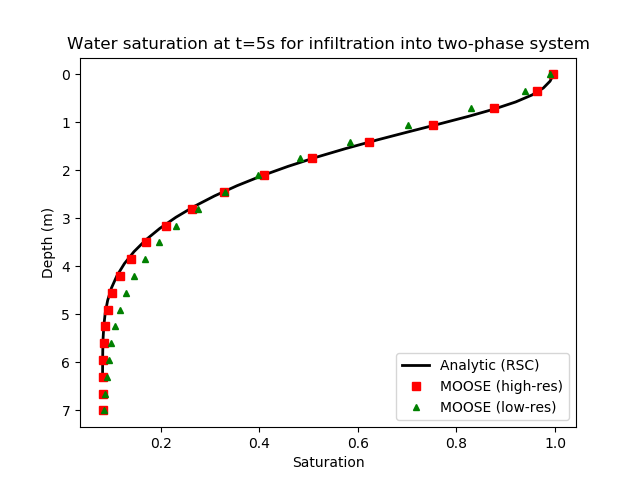Figure 6: Water saturation profile after 5 seconds of injection in the Rogers-Stallybrass-Clements test. The initial water saturation is 0.08181, and water is injected at the top of this figure at a constant rate. This forms a water front which displaces the oil. Black line: RSC's analytic solution. Red squares: high-resolution MOOSE simulation. Green triangles: lower resolution MOOSE simulation.

1. P. Broadbridge and I. White. Constant rate rainfall infiltration: a versatile nonlinear model, 1. analytical solution. Water Resources Research, 24:145–154, 1988.[BibTeX]
2. P. A. Forsyth, Y. S. Wu, and K. Pruess. Robust numerical methods for saturated-unsaturated flow with dry initial conditions in heterogeneous media. Water Resources Research, 18:25–38, 1995.[BibTeX]
3. C. Rogers, M. P. Stallybrass, and D. L. Clements. On two phase filtration under gravity and with boundary infiltration: application of a Backlund transformation. Nonlinear Analysis, Theory, Methods and Applications, 7:785–799, 1983.[BibTeX]
4. A. W. Warrick, D. O. Lomen, and A. Islas. An analytical solution to Richards' Equation for a Draining Soil Profile. Water Resources Research, 26:253–258, 1990.[BibTeX]